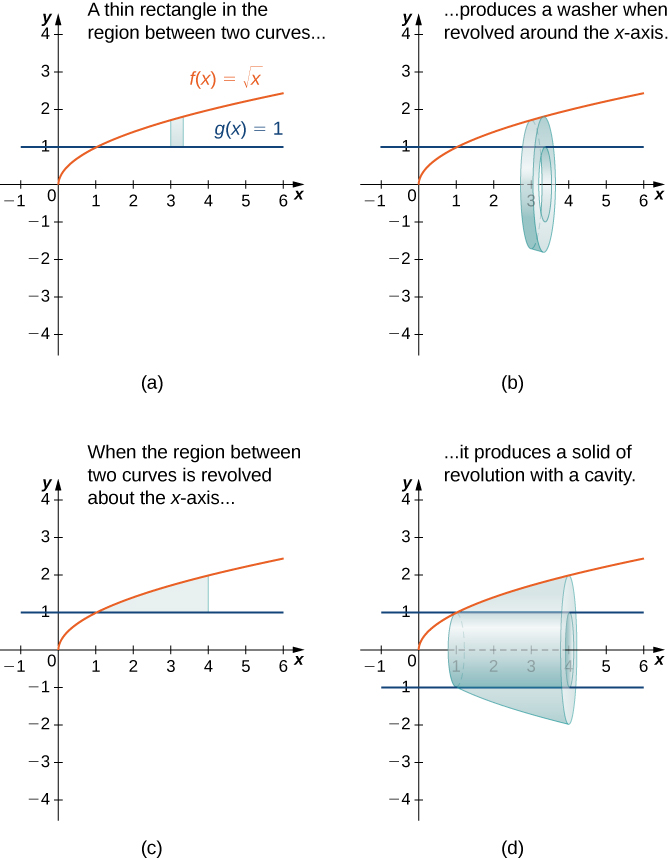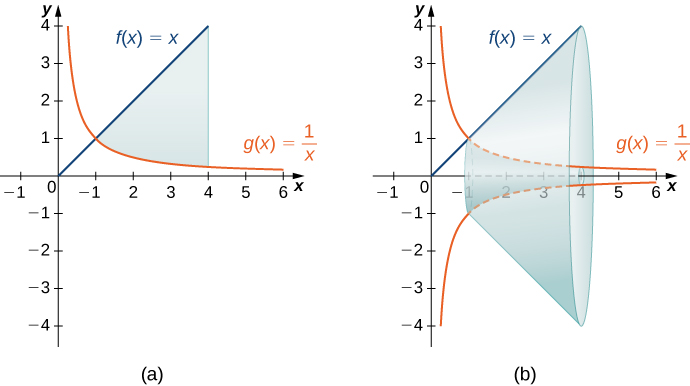# 6.2 Determining volumes by slicing  (Page 5/12)

 Page 5 / 12

## The washer method

Some solids of revolution have cavities in the middle; they are not solid all the way to the axis of revolution. Sometimes, this is just a result of the way the region of revolution is shaped with respect to the axis of revolution. In other cases, cavities arise when the region of revolution is defined as the region between the graphs of two functions. A third way this can happen is when an axis of revolution other than the $x\text{-axis}$ or $y\text{-axis}$ is selected.

When the solid of revolution has a cavity in the middle, the slices used to approximate the volume are not disks, but washers (disks with holes in the center). For example, consider the region bounded above by the graph of the function $f\left(x\right)=\sqrt{x}$ and below by the graph of the function $g\left(x\right)=1$ over the interval $\left[1,4\right].$ When this region is revolved around the $x\text{-axis,}$ the result is a solid with a cavity in the middle, and the slices are washers. The graph of the function and a representative washer are shown in [link] (a) and (b). The region of revolution and the resulting solid are shown in [link] (c) and (d).(a) A thin rectangle in the region between two curves. (b) A representative disk formed by revolving the rectangle about the x -axis . (c) The region between the curves over the given interval. (d) The resulting solid of revolution.

The cross-sectional area, then, is the area of the outer circle less the area of the inner circle. In this case,

$A\left(x\right)=\pi {\left(\sqrt{x}\right)}^{2}-\pi {\left(1\right)}^{2}=\pi \left(x-1\right).$

Then the volume of the solid is

$\begin{array}{cc}\hfill V& ={\int }_{a}^{b}A\left(x\right)dx\hfill \\ & ={\int }_{1}^{4}\pi \left(x-1\right)dx={\pi \left[\frac{{x}^{2}}{2}-x\right]\phantom{\rule{0.2em}{0ex}}|}_{1}^{4}=\frac{9}{2}\pi \phantom{\rule{0.2em}{0ex}}{\text{units}}^{3}.\hfill \end{array}$

Generalizing this process gives the washer method    .

## Rule: the washer method

Suppose $f\left(x\right)$ and $g\left(x\right)$ are continuous, nonnegative functions such that $f\left(x\right)\ge g\left(x\right)$ over $\left[a,b\right].$ Let $R$ denote the region bounded above by the graph of $f\left(x\right),$ below by the graph of $g\left(x\right),$ on the left by the line $x=a,$ and on the right by the line $x=b.$ Then, the volume of the solid of revolution formed by revolving $R$ around the $x\text{-axis}$ is given by

$V={\int }_{a}^{b}\pi \left[{\left(f\left(x\right)\right)}^{2}-{\left(g\left(x\right)\right)}^{2}\right]dx.$

## Using the washer method

Find the volume of a solid of revolution formed by revolving the region bounded above by the graph of $f\left(x\right)=x$ and below by the graph of $g\left(x\right)=1\text{/}x$ over the interval $\left[1,4\right]$ around the $x\text{-axis}\text{.}$

The graphs of the functions and the solid of revolution are shown in the following figure.(a) The region between the graphs of the functions f ( x ) = x and g ( x ) = 1 / x over the interval [ 1 , 4 ] . (b) Revolving the region about the x -axis generates a solid of revolution with a cavity in the middle.

We have

$\begin{array}{cc}\hfill V& ={\int }_{a}^{b}\pi \left[{\left(f\left(x\right)\right)}^{2}-{\left(g\left(x\right)\right)}^{2}\right]dx\hfill \\ & =\pi {\int }_{1}^{4}\left[{x}^{2}-{\left(\frac{1}{x}\right)}^{2}\right]dx\phantom{\rule{0.2em}{0ex}}\text{}\phantom{\rule{0.2em}{0ex}}={\pi \left[\frac{{x}^{3}}{3}+\frac{1}{x}\right]\phantom{\rule{0.2em}{0ex}}|}_{1}^{4}=\frac{81\pi }{4}\phantom{\rule{0.2em}{0ex}}{\text{units}}^{3}.\hfill \end{array}$

Find the volume of a solid of revolution formed by revolving the region bounded by the graphs of $f\left(x\right)=\sqrt{x}$ and $g\left(x\right)=1\text{/}x$ over the interval $\left[1,3\right]$ around the $x\text{-axis}\text{.}$

$\frac{10\pi }{3}$ units 3

As with the disk method, we can also apply the washer method to solids of revolution that result from revolving a region around the y -axis. In this case, the following rule applies.

## Rule: the washer method for solids of revolution around the y -axis

Suppose $u\left(y\right)$ and $v\left(y\right)$ are continuous, nonnegative functions such that $v\left(y\right)\le u\left(y\right)$ for $y\in \left[c,d\right].$ Let $Q$ denote the region bounded on the right by the graph of $u\left(y\right),$ on the left by the graph of $v\left(y\right),$ below by the line $y=c,$ and above by the line $y=d.$ Then, the volume of the solid of revolution formed by revolving $Q$ around the $y\text{-axis}$ is given by

$V={\int }_{c}^{d}\pi \left[{\left(u\left(y\right)\right)}^{2}-{\left(v\left(y\right)\right)}^{2}\right]dy.$

#### Questions & Answers

Find the derivative of g(x)=−3.
Abdullah Reply
any genius online ? I need help!!
Guzorochi Reply
how can i help you?
Pina
need to learn polynomial
Zakariya
i will teach...
nandu
I'm waiting
Zakariya
plz help me in question
Abish
evaluate the following computation (x³-8/x-2)
Murtala Reply
teach me how to solve the first law of calculus.
Uncle Reply
what is differentiation
Ibrahim Reply
f(x) = x-2 g(x) = 3x + 5 fog(x)? f(x)/g(x)
Naufal Reply
fog(x)= f(g(x)) = x-2 = 3x+5-2 = 3x+3 f(x)/g(x)= x-2/3x+5
diron
pweding paturo nsa calculus?
jimmy
how to use fundamental theorem to solve exponential
JULIA Reply
find the bounded area of the parabola y^2=4x and y=16x
Omar Reply
what is absolute value means?
Geo Reply
Chicken nuggets
Hugh
🐔
MM
🐔🦃 nuggets
MM
(mathematics) For a complex number a+bi, the principal square root of the sum of the squares of its real and imaginary parts, √a2+b2 . Denoted by | |. The absolute value |x| of a real number x is √x2 , which is equal to x if x is non-negative, and −x if x is negative.
Ismael
find integration of loge x
Game Reply
find the volume of a solid about the y-axis, x=0, x=1, y=0, y=7+x^3
Godwin Reply
how does this work
Brad Reply
Can calculus give the answers as same as other methods give in basic classes while solving the numericals?
Cosmos Reply
log tan (x/4+x/2)
Rohan
please answer
Rohan
y=(x^2 + 3x).(eipix)
Claudia
is this a answer
Ismael
A Function F(X)=Sinx+cosx is odd or even?
WIZARD Reply
neither
David
Neither
Lovuyiso
f(x)=1/1+x^2 |=[-3,1]
Yuliana Reply
apa itu?
fauzi

### Read also:

#### Get Jobilize Job Search Mobile App in your pocket Now!

Source:  OpenStax, Calculus volume 1. OpenStax CNX. Feb 05, 2016 Download for free at http://cnx.org/content/col11964/1.2
Google Play and the Google Play logo are trademarks of Google Inc.

Notification Switch

Would you like to follow the 'Calculus volume 1' conversation and receive update notifications?By OpenStaxBy Mackenzie WilcoxBy Saylor FoundationBy Stephen VoronBy RhodesBy OpenStaxBy Anindyo MukhopadhyayByBy OpenStaxBy OpenStax